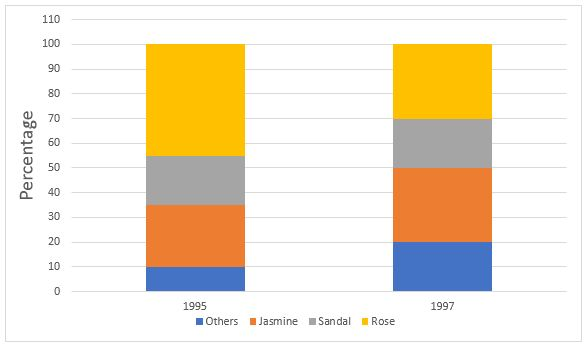## SSC CGL Quant Test 6

Instructions

The production figures of a perfume manufacturer are given in the form of percentage in sub-divided bar diagram. Study the diagram and answer the questions.Question 1

What is the ratio of percentage production of rose perfume during 1995 to that
during the year 1997 ?

Question 2

What is the percentage of production of sandal perfume during the year 1995 over
that during 1997 ?

Question 3

What is the production of jasmine perfume in the year 1997? Given that during the
year 1997 total perfumed production was 5000 units.

Instructions

For the following questions answer them individually

Question 4

If sinθ = 0.7, then cos θ, 0 ≤ θ < 90°, is

Question 5

Among the angles 30°, 36°, 45°, 50° one angle cannot be an exterior angle of a regular polygon. The angle is